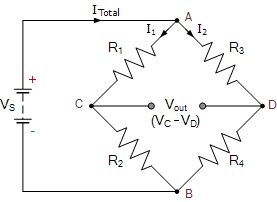# Working Principle of Sensors

A few sensor work on the bridge concept but every sensor has its own sensing element with some different phenomena such as physical principle, chemical principles etc. so let us understand the working principle of sensors.

### PHYSICAL PRINCIPLES

1. Ampere’s Law
• A current-carrying conductor in a magnetic field experiences a force (e.g. galvanometer)
2. Curie-Weiss Law
• There is a transition temperature at which ferromagnetic materials exhibit paramagnetic behavior
• A coil resist a change in the magnetic field by generating an opposing voltage/current (e.g. transformer)
4. Photoconductive Effect
• When light strikes certain semiconductor materials, the resistance of the material decreases (e.g. photoresistor)

### TO CLARIFY BRIDGE CONCEPT SEE FIGURE BELOW

In this circuit we have a battery of 12V and the total current ITotal is flowing.

• A bridge circuit or two branches in parallel are connected at two terminals A and B of the battery end.
• Bridge circuit contains four resistors R1, R2, R3 and R4. And there are four points A, B, C and D.

Total current ITotal is being divided at point A in current I1 and I2 and at point B  total current is I1 + I2 = ITotal. Current I1 follows path ACB and current I2 follows path ADB.Now, we know the current follows a low resistive path. If the resistance R1 = R3 and R2 = R4 that means the value of resistance is the same in both paths so I1 will equal to I2 (i.e. I1 = I2).

That means Voltage drop at R1 and R3 are the same, so the voltage at points C and D are the same which means potential difference (voltage) between point C and D is zero (VC – VD = 0) this is known as balanced bridge network.

If the value of resistance changes in any path can make a change in potential difference which will be measured and this is known as an unbalanced bridge network.

So if we replace any resistor with any sensor for example replace R2 with LDR (Light-dependent resistor) or any variable resistor. Then as the value of resistor changes either increases or decreases,

• If resistance increases in path ACB, the voltage drop is higher in path ACB and lesser current will pass through path ACB and
• If resistance increases in path ADB, the voltage drop is higher in path ADB and lesser current will pass through path ADB.

So we can have both values of the result either positive or negative to detect changes.

### WE CAN PROOF WORKING PRINCIPLE OF SENSORS WITH AN EXAMPLE

See bridge circuit below

CONDITION 1

As both the resistors are in series, current (I) flows through both of them is the same. Therefore, the current (I) is given as V/RT

I = V ÷ R => 12V ÷ (10Ω + 20Ω) => 0.4AThe voltage at point C (which is remaining voltage left after dropped at R1 i.e. 12 – 4 = 8), which is also the voltage drop across the lower resistor, R2 is calculated as:

VR2 = I × R2 = 0.4A × 20Ω = 8 V

Thus we can see that the source voltage VS is divided among the two series resistors in direct proportion to their resistances as VR1 = 4V and VR2 = 8V.

This is the principle of voltage division, also known as a voltage divider circuit or network.

#### CONDITION 2

Now if we add another series resistor circuit using the same resistor values in parallel with the first (Voltage in the parallel branch is same), we  have the resultant circuit like thisAs the second series circuit has the same resistance values of the first, the voltage at point D, which is also the voltage drop across the resistor, R4 will be the same 8 volts, with respect to ground (battery negative), as the voltage is common and the two resistive networks are the same.

But something is also important, that the voltage (potential difference) between point C and point D will be zero volts as both points are at the same value of 8 volts as: C = D = 8 volts, then the voltage (potential difference) is: 0 volts

When the voltage at point C is the same value as the voltage at point D or their potential difference is zero then the bridge network is said to be balanced.

#### CONDITION 3

Now let’s consider what happens if we invert the position of resistors R3 and R4 in the second parallel branch with respect to R1 and R2.

The current flows through the series resistor combination is same but the voltage at point D, which is also the voltage drop across the resistor, R4 will be:

VR4 = 0.4A × 10Ω = 4 volts

Now with VR4 having 4 volts dropped across it, the voltage (potential difference) between points C and D is 4 volts as: C = 8 volts and D = 4 volts. Now the potential difference this time is: 8 – 4 = 4 voltsThe result of changing resistance in any parallel branch gives us the positive or negative potential difference between points C and D which is said to be an unbalanced network.

The resistance ratio of these two parallel arms, ACB and ADB, results in a potential difference, lies between 0 volts (balanced) and the maximum supply voltage (unbalanced), and this is the basic principle of a bridge circuit.

As we learn working principle of sensors now let add on with LDR in this circuit for making darkness sensor circuit.

LDR – LED ON AS LIGHT FALLS